# Understanding Price Elasticity of Demand: Definition, Formula, and More

Last updated: 03 Dec 2021

## What Is Price Elasticity?

Price Elasticity (more formally, Price Elasticity of Demand) is a measure of how strongly buyers react to changes in price.

Because quantity purchased usually goes down when price increases, the price elasticity for a good (or service) is usually negative. But, the rate at which quantity purchased goes down can vary a lot from one product category to another, for different market segments, due to the degree of competition, and from one brand to another.

The degree of price elasticity (pricing sensitivity) affecting your product(s) makes a big difference to your marketing and pricing efforts.

### Formula for Elasticity of Demand

The common formula for price elasticity is:

``` %Change in Quantity Demanded / %Change in Price. ```

For example, imagine that a firm sells 1000 units during time period 0 at a price of \$100. In time period 1, the firm raises its price by 10% to \$110 and achieves sales of 950 units (a loss of 5% in quantity demanded).

The price elasticity of demand for the firm is -5%/10% = -0.5. Note that due to the price increase the firm’s revenue increases to \$110 x 950 = \$104,500 in time period 1 from \$100 x 1000 = \$100,000 in time period 0.

## Why Does Pricing Elasticity Matter?

One of the key roles of marketing is to reduce the price elasticity for the firm’s offerings which (when price is set properly) leads to increased profits.

Firms do so by (among other things) raising the perception of the brand, improving the brand’s features, or improving reliability. Thus, regularly measuring price elasticity via a method such as conjoint analysis can assist in brand management and in tracking changes to brand equity.

Commodities like wheat and corn are considered substitutable and the price elasticity is relatively high.

Other products like luxury vehicles will have lower price elasticity, since they are not viewed as interchangeable as wheat and their buyers typically have relatively high incomes.

## Understanding Price Elasticity of Demand

Price Elasticity Description Typical Strategy
-∞ to < -1 Elastic: changes in price are matched by a stronger change in quantity demanded Decrease price to increase revenues
-1 Unitary Elastic: changes in price are matched by an equal change in quantity demanded Depends on the firm’s desire to increase production or not to achieve higher share
> -1 to 0 Inelastic: changes in price are matched by a weaker change in quantity demanded Increase price to increase revenues
> 0 to ∞ Giffen good: increases in price are associated with increases in quantity demanded (and vice-versa) Increase prices to increase revenues, subject to price thresholds

It’s important to note that price elasticity usually depends on the starting price point along the price curve. In other words, the price elasticity associated with making a 10% price increase on a product currently at \$100 is often different from the price elasticity associated with a 10% price increase if the product is currently at \$120. This point is illustrated in the chart below: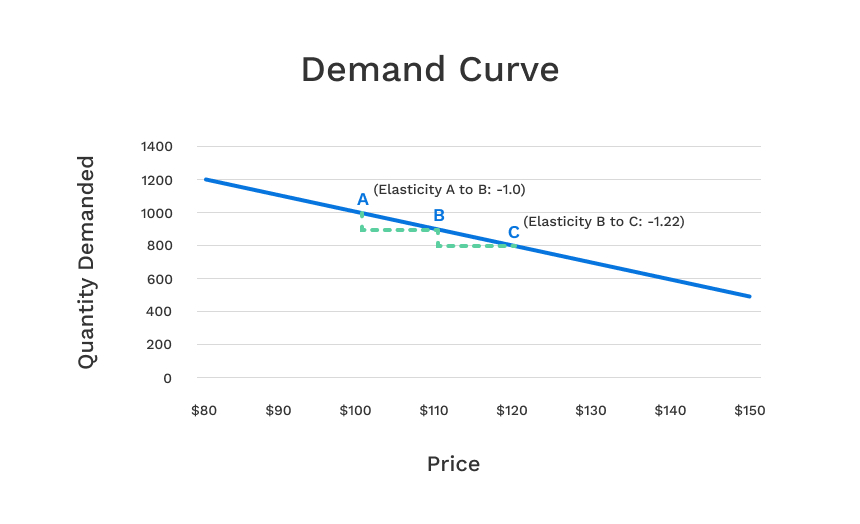(Note that when using conjoint analysis to derive demand curves, we’re typically using sensitivity analysis in a market simulator to find the share of preference at each price point. Thus, we’d plot share of preference on the Y-axis instead of Quantity Demanded.)

In the above simplified demand curve, the quantity demanded has a linear relationship with price. For illustration, each \$10 increase in price is associated with a 100-unit decrease in quantity demanded.

At starting point A, if we increase price by 10% from \$100 to \$110, the quantity demanded falls by 10% from 1000 to 900 units, as shown at point B. Thus, the price elasticity is -1.0 when considering the price change from A to B. However, increasing the price from \$110 to \$120 (from points B to C, a 9.09% increase in price) leads to an 11.1% decrease in quantity demanded, for a price elasticity of -1.22.

This leads to the important observation that linear demand curves have different price elasticity at each point along the curve.

A curvilinear demand curve can be made to have constant price elasticity at every price point, as in the example below: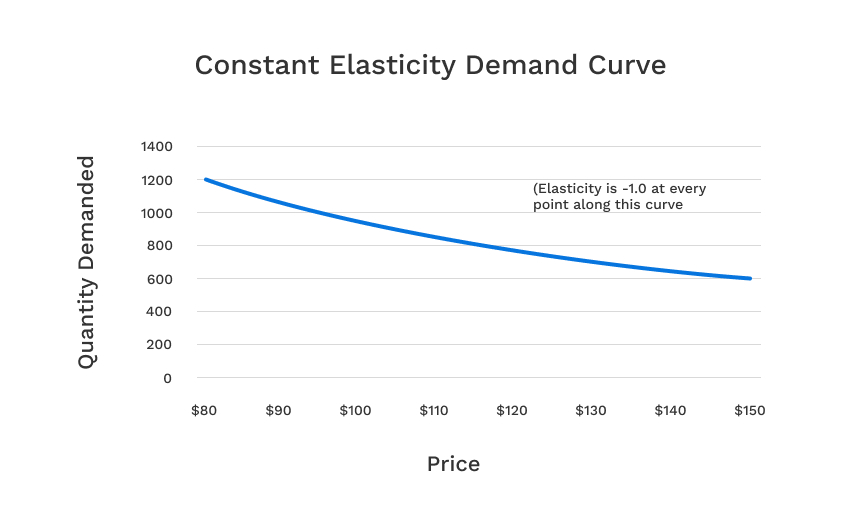Another important insight when interpreting demand curves is that increases in demand (a shift higher in the demand curve) generally lead to lower price elasticities (and vice-versa):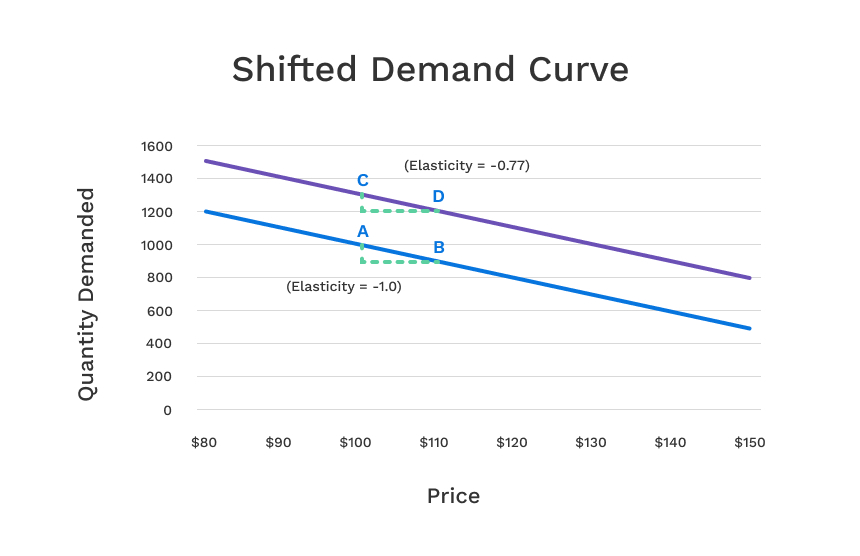In the example above, the two demand curves are parallel, and yet the elasticity from point A to point B is -1.0, while the elasticity from point C to D on the higher demand curve is -0.77. A shifted upward demand curve represents a superior brand equity position.

## When Price Elasticity Is Positive

In rare situations, some goods have positive elasticity over a given price range, meaning that quantity increases with increases in price (called Giffen goods), and vice-versa.

More realistically, we sometimes find (using conjoint analysis) a portion of the demand curve exhibiting positive elasticity, followed by a point at which the demand curve takes on the usual down-sloping shape.

We recently saw this in a conjoint study involving purchase of homes, where pricing the home too low can result in lower share of preference: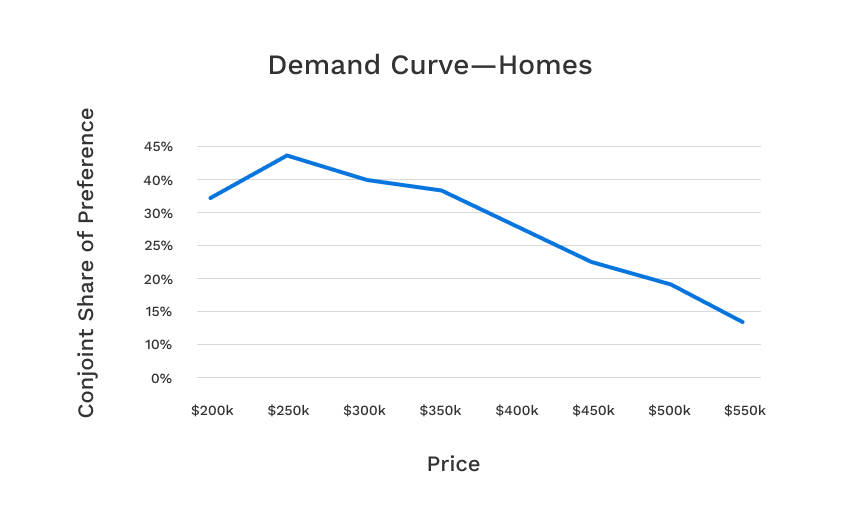## How to Calculate Pricing Elasticity (3 Common Ways)

Simple elasticity formula considering one starting price point and one direction of change (either up or down):

``` %Change in Quantity Demanded / %Change in Price ```

Midpoints formula (Arc elasticity—the average elasticity between two price points):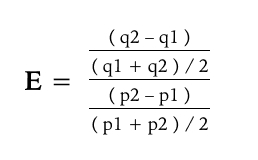Where: p1 and p2 are price points, and q1 and q2 are quantity demanded associated with those two price points.

Log-log regression (computes the average elasticity over the range of prices):

Often when using conjoint analysis we use a market simulator to estimate the price and quantity demanded (share of preference) coordinates over a range of prices. When we have these data, we often want to summarize the average price elasticity per brand across the range:

Price Brand A
Quantity Demanded
(or share of preference)
Brand B
Quantity Demanded
(or share of preference)
\$100 824 1231
\$110 726 1057
\$125 651 910
\$150 599 804

(Note that share of preference is the most common output used from conjoint analysis as a proxy for quantity demanded.)

We first transform the data by taking the natural log of prices and the natural log of quantity demanded:

Ln(Price) Brand A
Ln(Quantity Demanded)
Brand B
Ln(Quantity Demanded)
4.605 6.714 7.116
4.700 6.588 6.963
4.828 6.479 6.813
5.011 6.395 6.690

Next, we compute two linear regressions (one for Brand A and one for Brand B) using the transformed table above, predicting Ln(quantity demanded) for each brand using Ln(Price) as the independent variable. The slope (beta estimate) of Ln(Price) predicting Ln(quantity demanded) is the average price elasticity of demand across the range. For the data above, the elasticities (the regression weights) using the log-log regression are Brand A: -0.765 and Brand B: -1.031.

## Estimating Price Elasticity from Sales Data

Sometimes, you’re able to estimate price elasticity with sales data. Models such as logistic regression may be applied. However, efforts to do this often run into severe roadblocks, including: not enough independent variation in the prices across your firm and competitors and differences in prices across regions of the marketplace.

## Estimating Pricing Sensitivity Using Surveys

Price elasticity can be estimated in survey-based research using conjoint analysis. Other common, though typically less effective survey-based methods for estimating price elasticity include Van Westendorp Price Sensitivity Meter (with the Newton-Miller-Smith extension) and Gabor-Granger.